Examples

Chapter 11 Class 9 Constructions [Deleted]
Serial order wise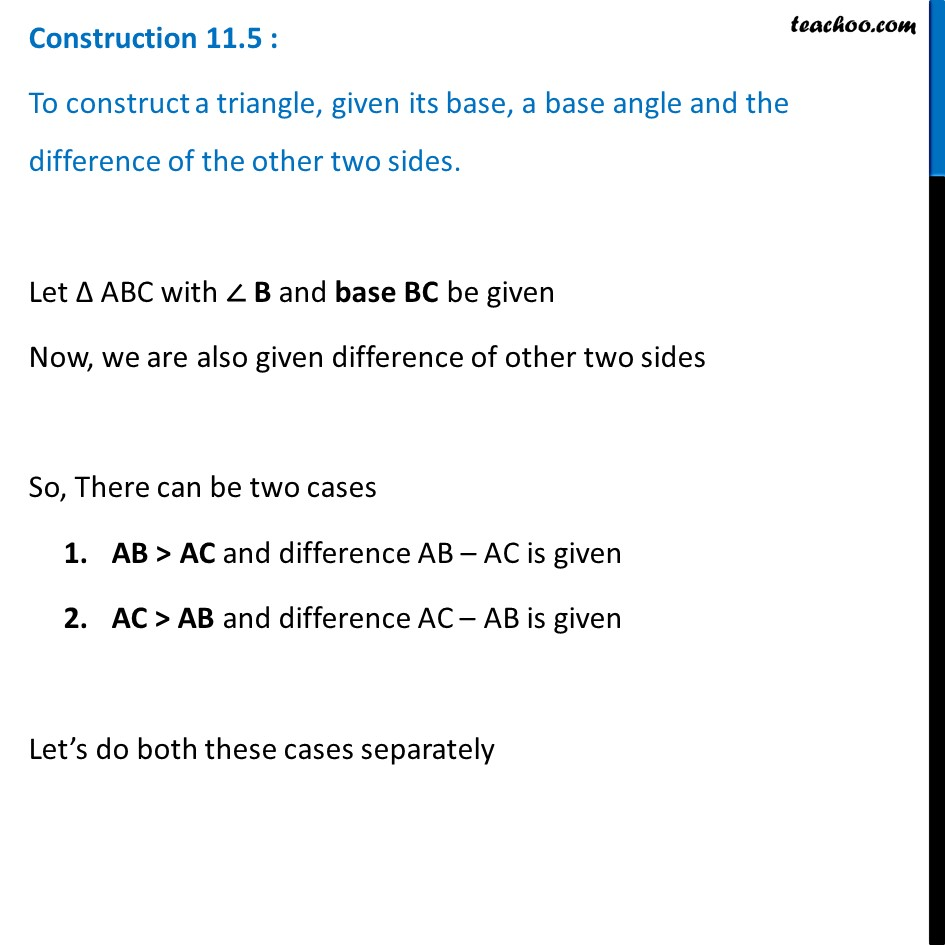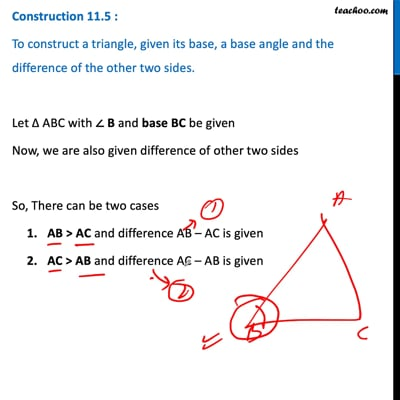This video is only available for Teachoo black users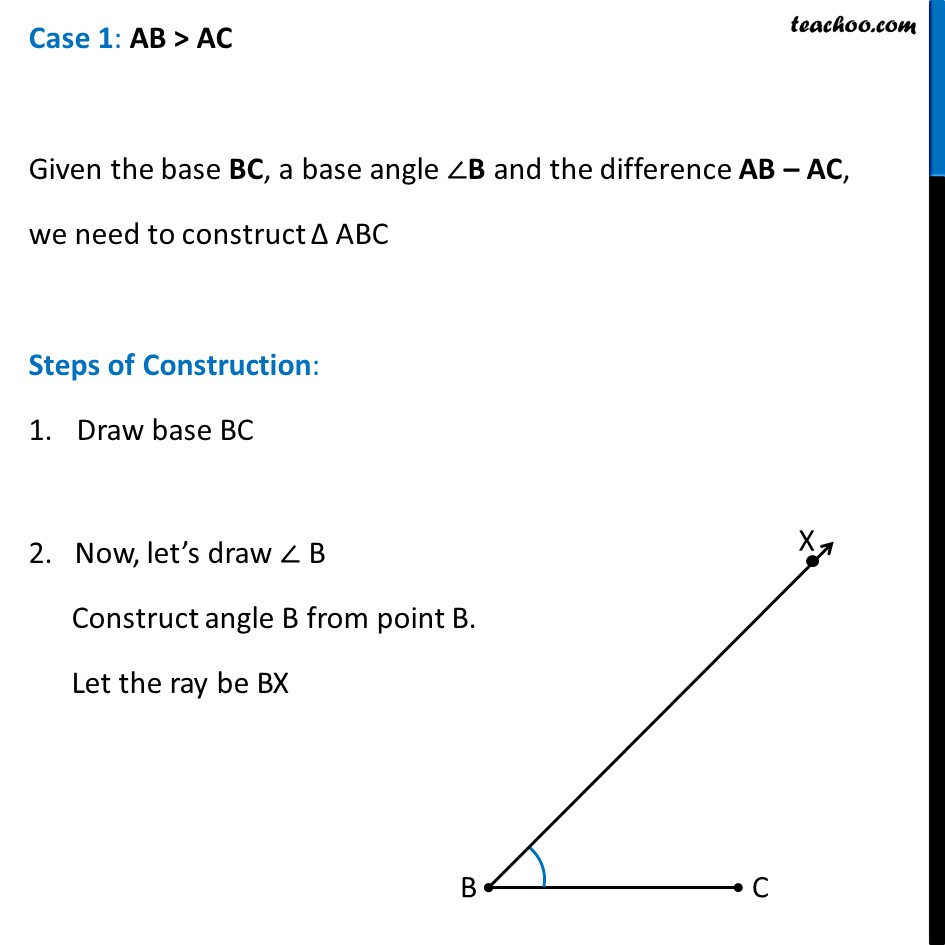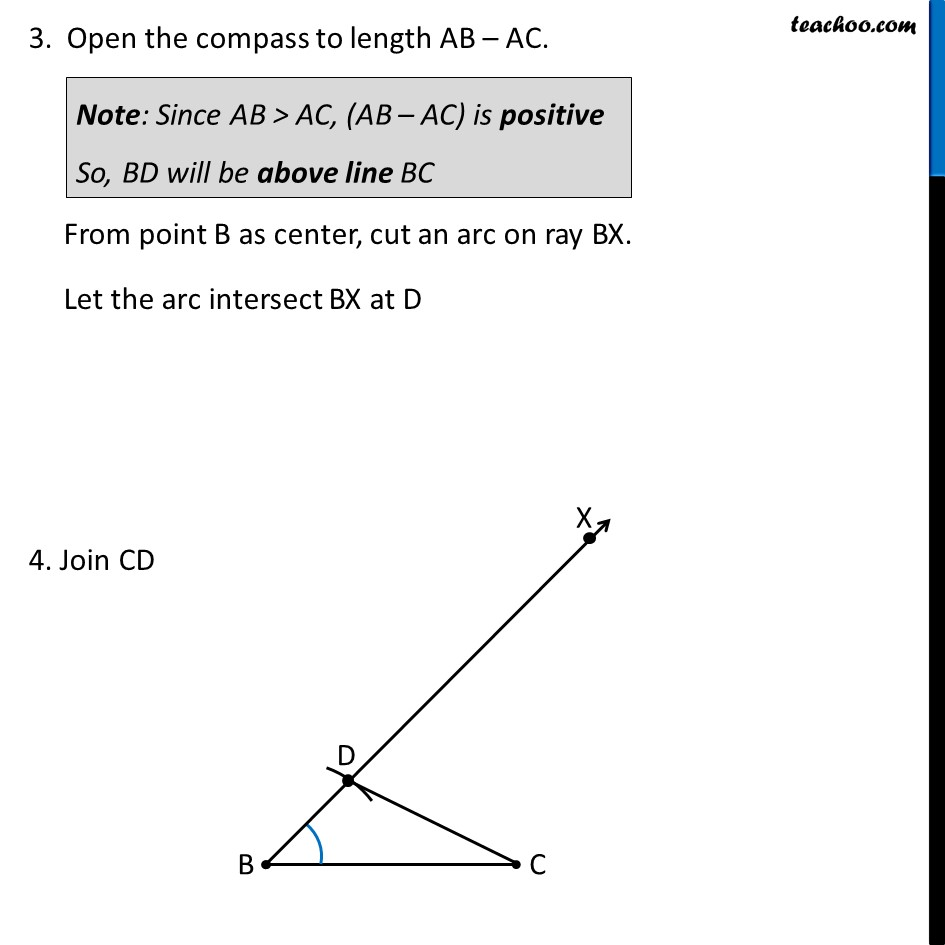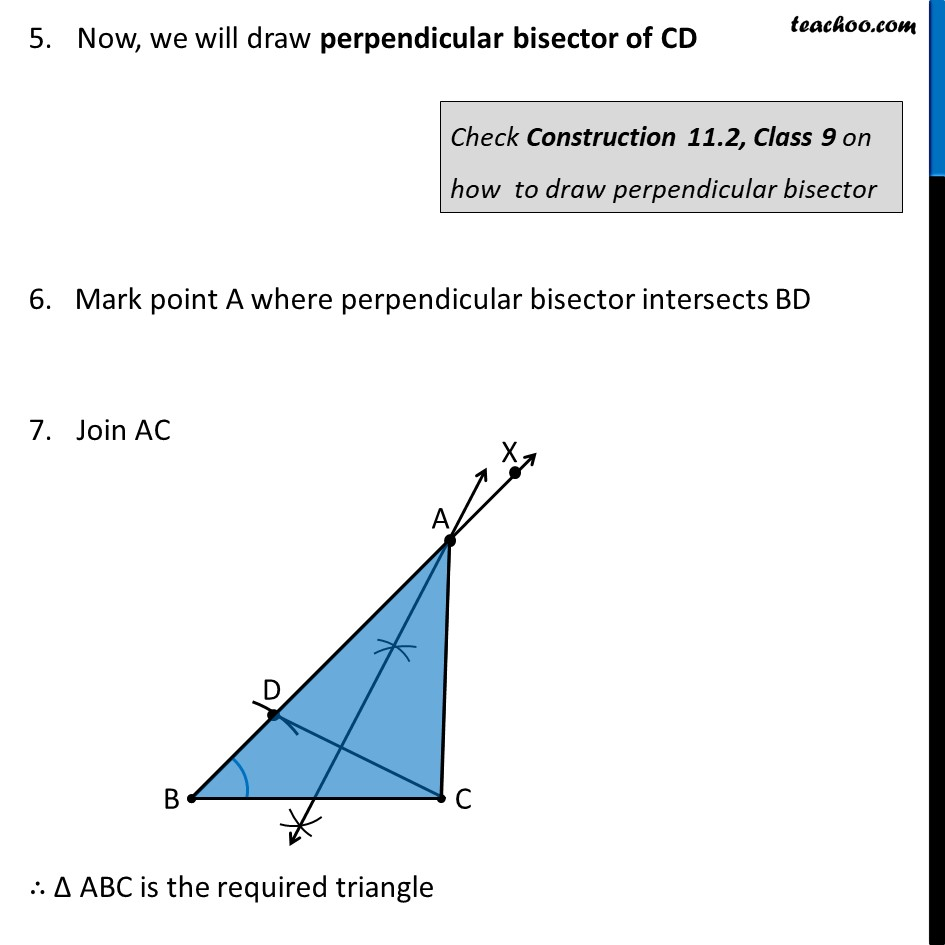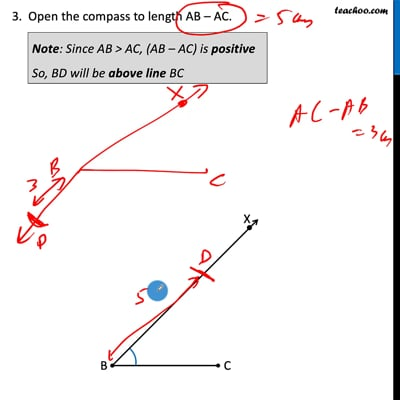This video is only available for Teachoo black users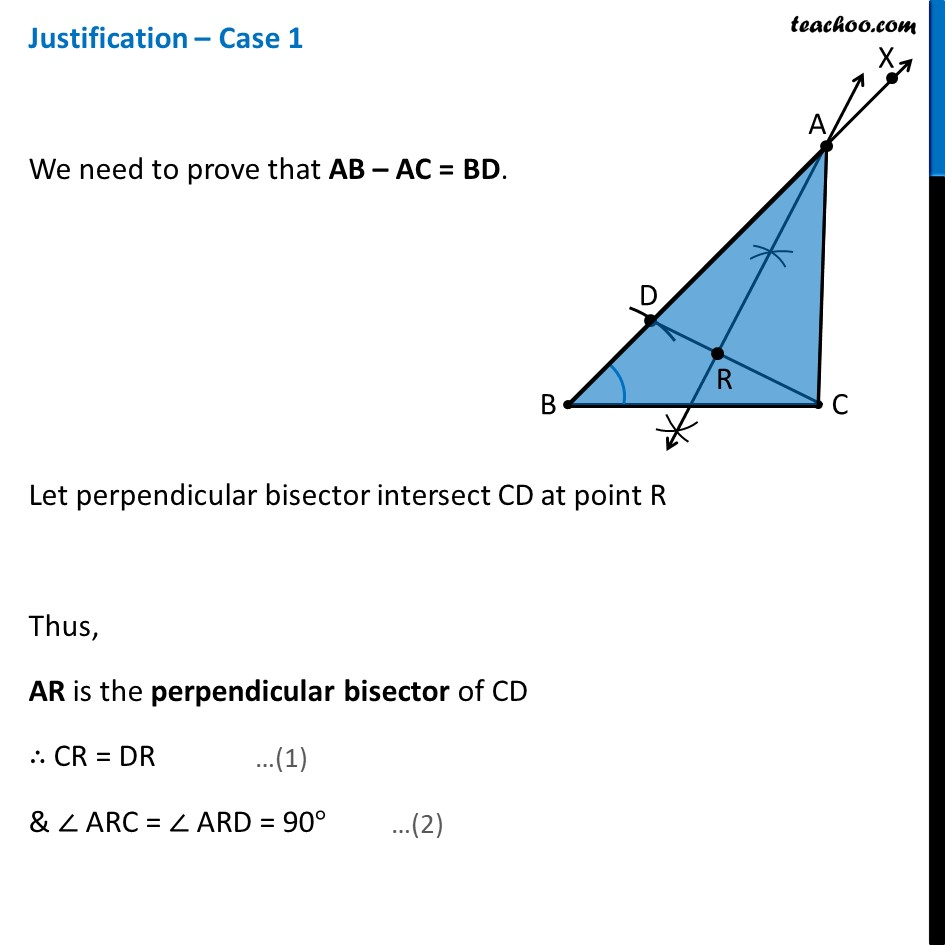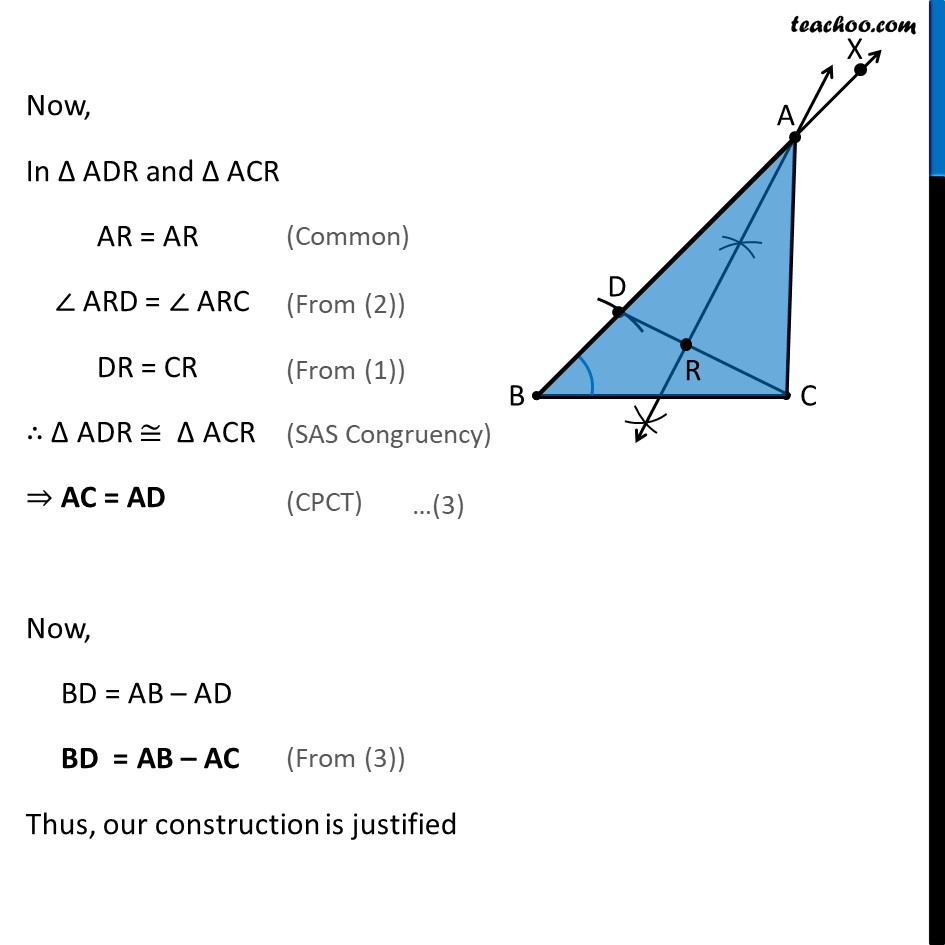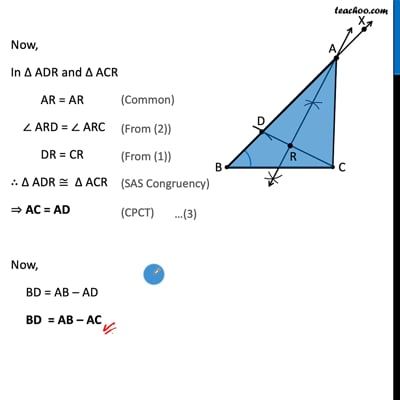This video is only available for Teachoo black users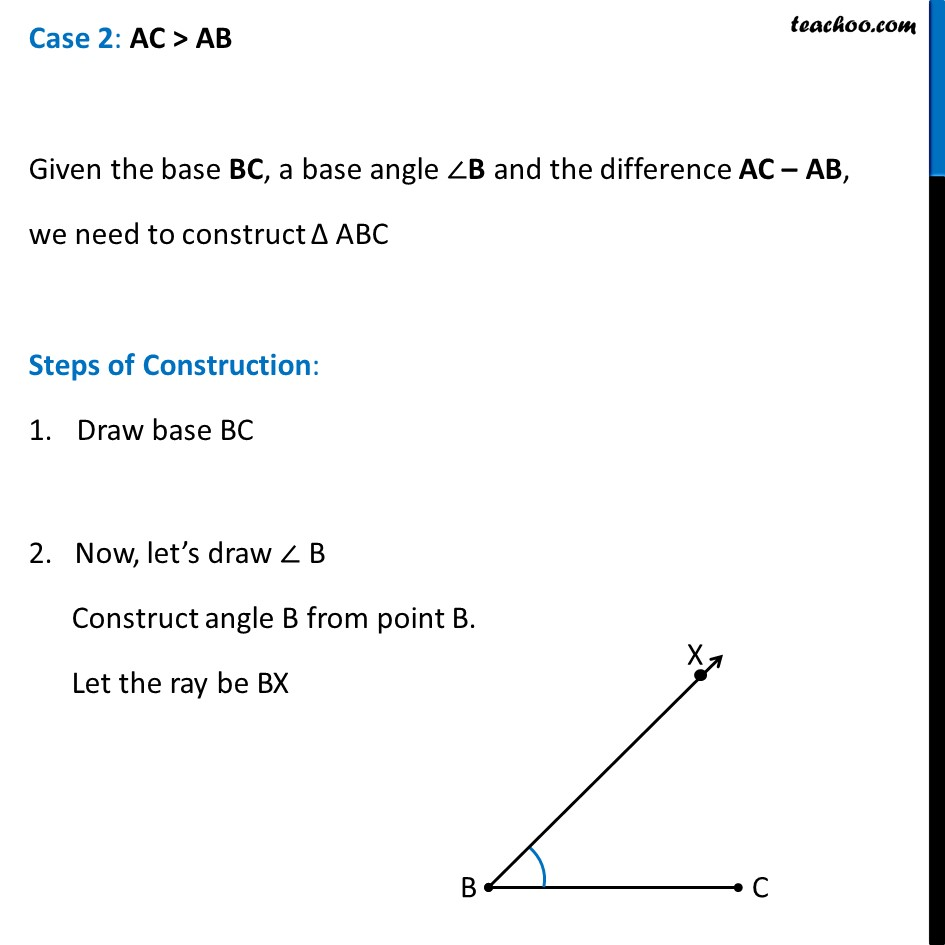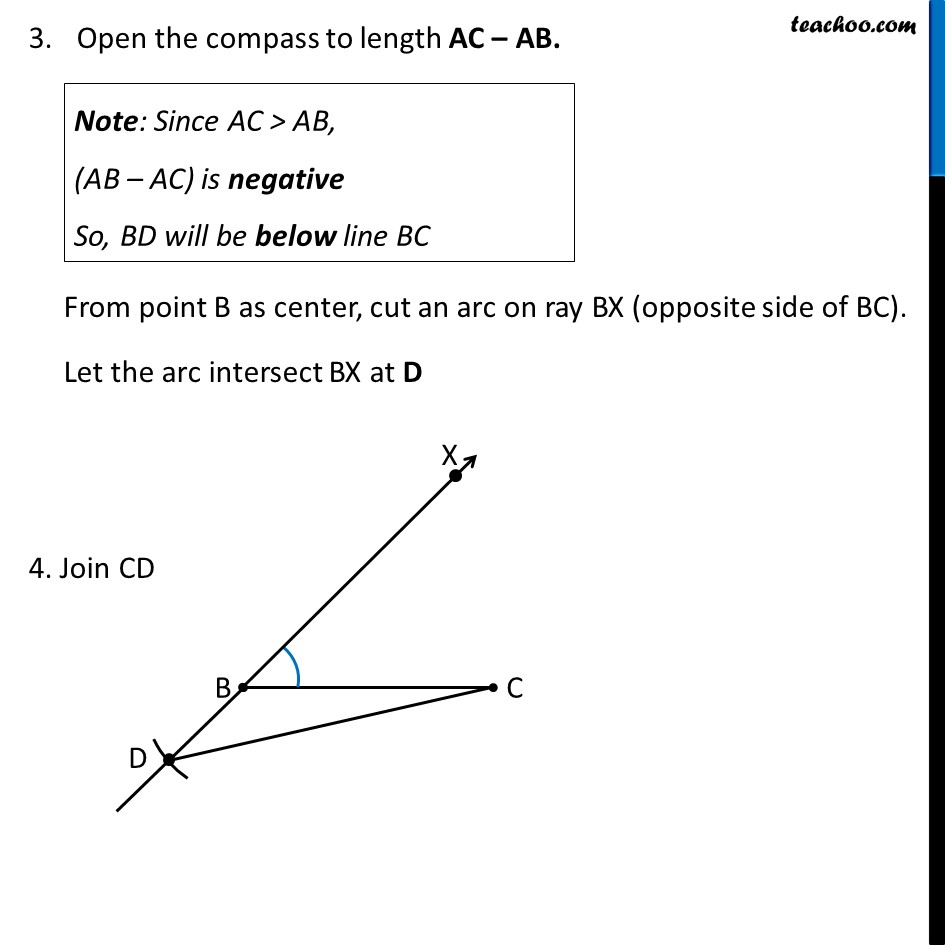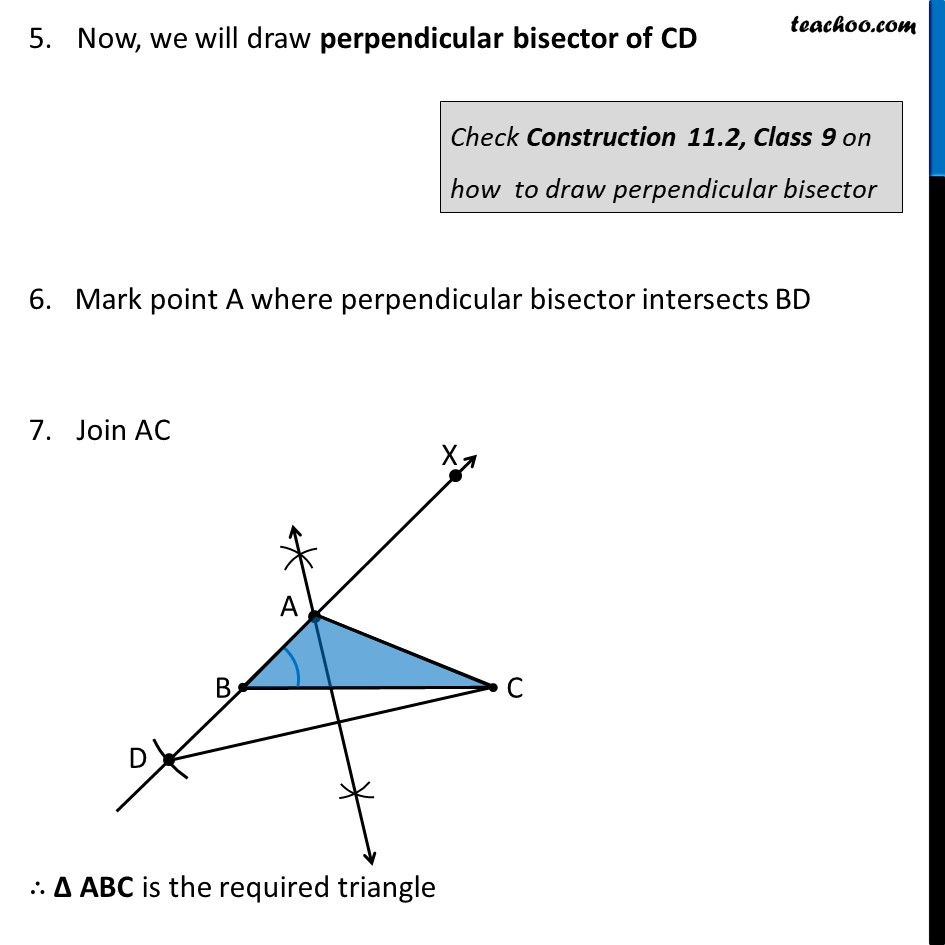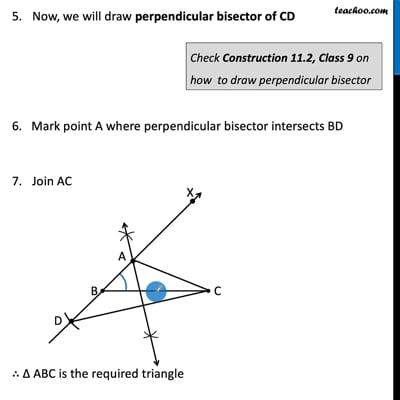This video is only available for Teachoo black users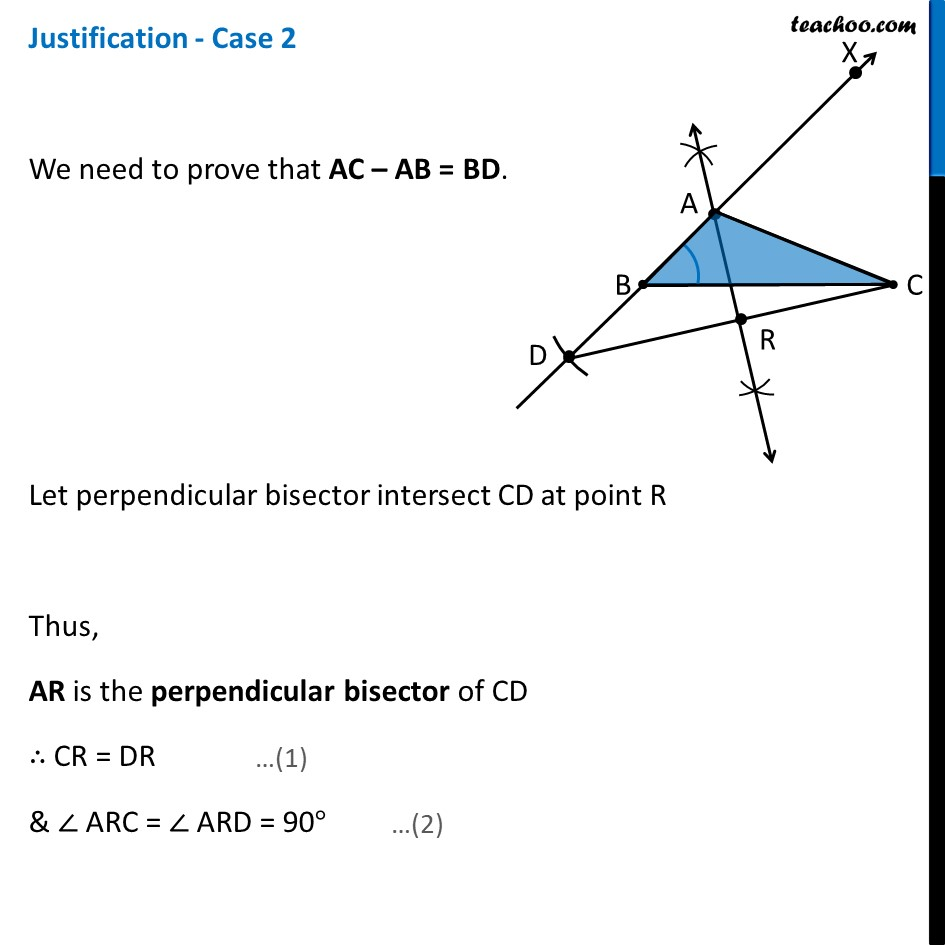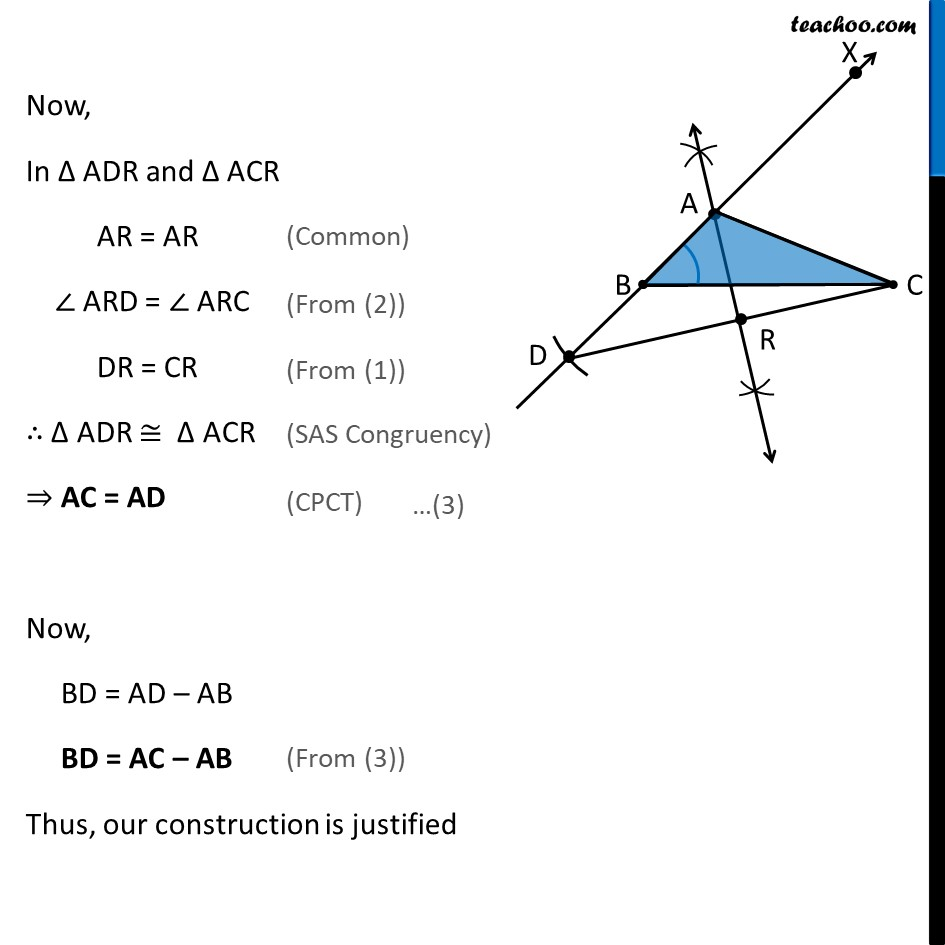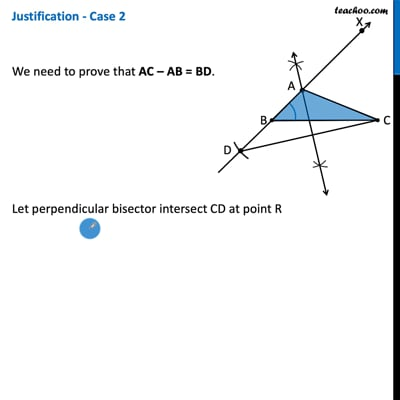This video is only available for Teachoo black users

Maths Crash Course - Live lectures + all videos + Real time Doubt solving!

### Transcript

Construction 11.5 : To construct a triangle, given its base, a base angle and the difference of the other two sides. Let Δ ABC with ∠ B and base BC be given Now, we are also given difference of other two sides So, There can be two cases AB > AC and difference AB – AC is given AC > AB and difference AC – AB is given Let’s do both these cases separately Case 1: AB > AC Given the base BC, a base angle ∠B and the difference AB – AC, we need to construct Δ ABC Steps of Construction: Draw base BC 2. Now, let’s draw ∠ B Construct angle B from point B. Let the ray be BX 3. Open the compass to length AB – AC. From point B as center, cut an arc on ray BX. Let the arc intersect BX at D 4. Join CD Note: Since AB > AC, (AB – AC) is positive So, BD will be above line BC Now, we will draw perpendicular bisector of CD 6. Mark point A where perpendicular bisector intersects BD Join AC ∴ Δ ABC is the required triangle Check Construction 11.2, Class 9 on how to draw perpendicular bisector Justification – Case 1 We need to prove that AB – AC = BD. Let perpendicular bisector intersect CD at point R Thus, AR is the perpendicular bisector of CD ∴ CR = DR & ∠ ARC = ∠ ARD = 90° Now, In Δ ADR and Δ ACR AR = AR ∠ ARD = ∠ ARC DR = CR ∴ Δ ADR ≅ Δ ACR ⇒ AC = AD Now, BD = AB – AD BD = AB – AC Thus, our construction is justified (Common) (From (2)) (From (1)) (SAS Congruency) (CPCT) (From (3)) Case 2: AC > AB Given the base BC, a base angle ∠B and the difference AC – AB, we need to construct Δ ABC Steps of Construction: Draw base BC 2. Now, let’s draw ∠ B Construct angle B from point B. Let the ray be BX Open the compass to length AC – AB. From point B as center, cut an arc on ray BX (opposite side of BC). Let the arc intersect BX at D 4. Join CD Note: Since AC > AB, (AB – AC) is negative So, BD will be below line BC Now, we will draw perpendicular bisector of CD 6. Mark point A where perpendicular bisector intersects BD Join AC ∴ Δ ABC is the required triangle Check Construction 11.2, Class 9 on how to draw perpendicular bisector Justification - Case 2 We need to prove that AC – AB = BD. Let perpendicular bisector intersect CD at point R Thus, AR is the perpendicular bisector of CD ∴ CR = DR & ∠ ARC = ∠ ARD = 90° Now, In Δ ADR and Δ ACR AR = AR ∠ ARD = ∠ ARC DR = CR ∴ Δ ADR ≅ Δ ACR ⇒ AC = AD Now, BD = AD – AB BD = AC – AB Thus, our construction is justified (Common) (From (2)) (From (1)) (SAS Congruency) (CPCT)